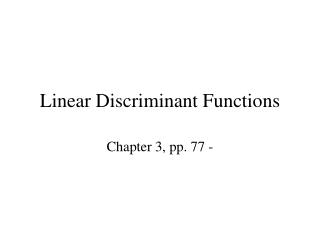DownloadDownload PresentationLinear Discriminant Functions

# Linear Discriminant Functions

Download Presentation## Linear Discriminant Functions

- - - - - - - - - - - - - - - - - - - - - - - - - - - E N D - - - - - - - - - - - - - - - - - - - - - - - - - - -
##### Presentation Transcript

1. Linear Discriminant Functions Chapter 3, pp. 77 -

2. Linear Discriminant Functions • Chapter 1 introduced the concept of a discriminant function y(x) • The vector x is assigned to class C1 if y(x)>0 and C2 if y(x)<0. • Simplest choice of such a function is linear in the components of x, and therefore can be written as

3. terminology • w is weight vector, d-dimensional • w0 is bias, -w0 is threshold

4. Geometric interpretation of (3.1) • Decision boundary y(x)=0 corresponds to (d-1)-dimensional hyperplane in d-dimensional x-space. • For d=2 (plane), decision boundary is a straight line

5. Geometry (cont’d) • If xA and xB are 2 pts on the hyperplane, then y(xA) and y(xB) are 0. • So, using (3.1), we have Thus w is normal to any vector lying in the hyperplane!

6. More on the nature of the hyperplane • We’ve seen that w is normal to any vector lying in the hyperplane! • Thus w determines the orientation of the hyperplane • But how far is the hyperplane to the origin? • If x is any point on the hyperplane, then the normal dist from the origin to the hyperplane is… So the bias w0 determines the position of hyperplane

7. Classifying several classes • For each class Ck, define the discriminant function • A new point x is then assigned to class Ck if

8. How far is the classification boundary from the origin? • The boundary separating class Ck from class Cj is given by • Which correspond to (partial) hyperplanes of the form • By analogy to the 2-class case, the perpendicular distance of the decision boundary from the origin is given by

9. Expressing multiclass linear discriminat function as a neural network diagram